Measurements from the real world often contain noise. Loosely speaking, noise is just the part of the signal you didn’t want. Maybe it comes from electrical noise: the random variations you see when calling `analogRead` on a sensor that should be stable. Noise also arises from real effects on the sensor. Vibration from the engine adds noise if you’re measuring the acceleration of a go-kart, for example. Filtering is a method to remove some of the unwanted signal to leave a smoother result.

## Filters

We’ll use MegunoLink to compare three different filters:

1. Averaging
2. Running average
3. Expotential filter

MegunoLink’s Time Plot Visualizer will be used to show both the raw, unfiltered, data and the output from the filter. Comparing the raw and filtered on a single plot lets us easily see the effect of the filter. The filters will smooth the data but they can also introduce a lag.

## Something to Filter

To generate some ‘noisy’ data for filtering a thermistor was connected to analog-input 0 on an Arduino Uno. A thermistor is a resistor whose resistance changes with temperature. As temperature increases, resistance goes down; as temperature decreases, resistance goes up. The thermistor was connected in a voltage divider configuration with one leg connected to ground and the other to the analog-input. A 100kΩ resistance was connected between the 3.3V output of the Arduino and the analog-input to provide current for the thermistor.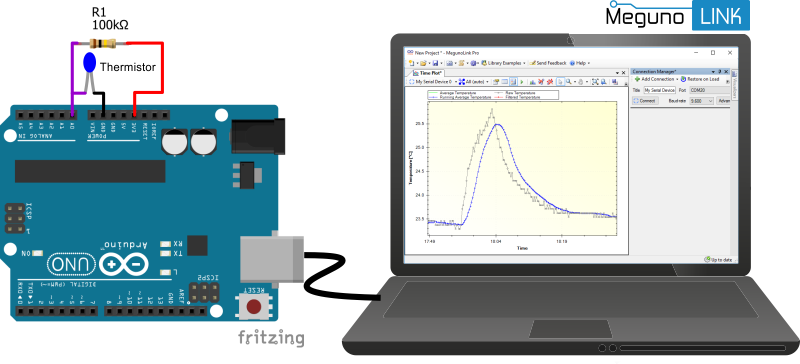The temperature measured using a thermistor connected to an Arduino was plotted in MegunoLink. Different filters were compared to see which one smoothed the temperature measurements best.

To measure temperature, the analog value was read. Then the raw analog value was converted to temperature, in degrees Celsius, using the following Arduino code:

After applying the various filters, the data was sent to MegunoLink for plotting:

## Filtering Algorithms

The three filtering algorithms we are going to look at are:

1. Averaging
2. Running average
3. Exponential filter

The screenshot from MegunoLink below shows these algorithms together. A small rise in temperature was created by touching the thermistor to see how the different filters would respond. The grey line is the raw temperature measurement. It is pretty good with not much noise. But there is still room for improvement.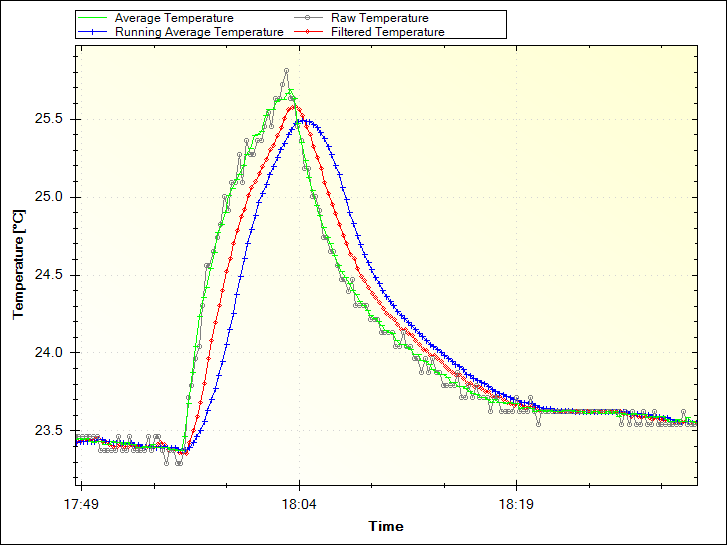The raw temperature signal (grey) smoothed by averaging 16 measurements (green), a running average of 16 measurements (blue) and an exponential recursive filter (red) is plotted. The temperature increase was caused by touching the thermistor.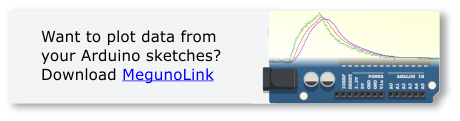### Averaging

One of the easiest ways to filter noisy data is by averaging.

Averaging works by adding together a number of measurements, the dividing the total by the number of measurements you added together. The more measurements you include in the average the more noise gets removed. There is a diminishing return though: the average of 101 measurements isn’t going to be much less noisy than the average of 100 measurements.

Here’s the code to calculate an average temperature measurement:

Notice the delay between each measurement. That delay is to give the analog-input time to stabilize between each measurement. Without it, your average will tend to be lower than the true measurement.

The picture below shows just the raw data and the average filter. Averaging, even only 16 measurements, does a pretty good job of smoothing the small random noise in the raw measurements. Even better, it tracks the change in temperature (when I touched the sensor) very closely.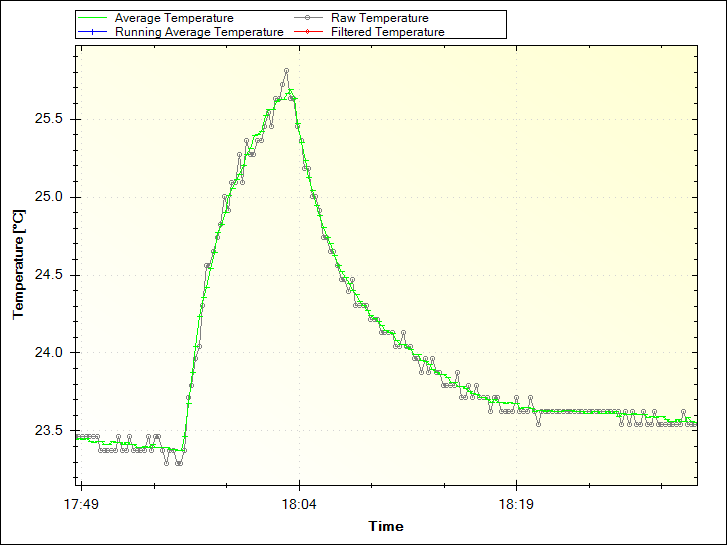A 16 measurement average (green) along with a single raw temperature measurement (grey) is plotted. Note how the average is smoother.

The average filter might be the best one for this application, when the original measurement is not very noisy.

### Running Average

One downside of the average filter is the amount of time needed to make a measurement. The measurement time can be important in low-power applications. The Arduino uses much more power when it is awake and running your program than it does when it asleep in standby.

An alternative to making all the measurements at once then averaging them is to make one measurement at a time and add it to a running average. The calculation of the average is the same: sum up all the measurements and divide by how many you made.

But we have to store the measurement history. Here’s a snippet of code to do the running average:

This gives a much slower response to changes because of the 100 ms delay between making each measurement. In other words, it only takes 16 ms for the average filter to get 16 new measurements but it takes 1.6 seconds (16 x 100 ms) for the running average to get 16 new measurements. So it responds to changes more slowly. And you can see that in the picture below. The grey line is the same raw temperature measurement as before. The running average is shown in blue.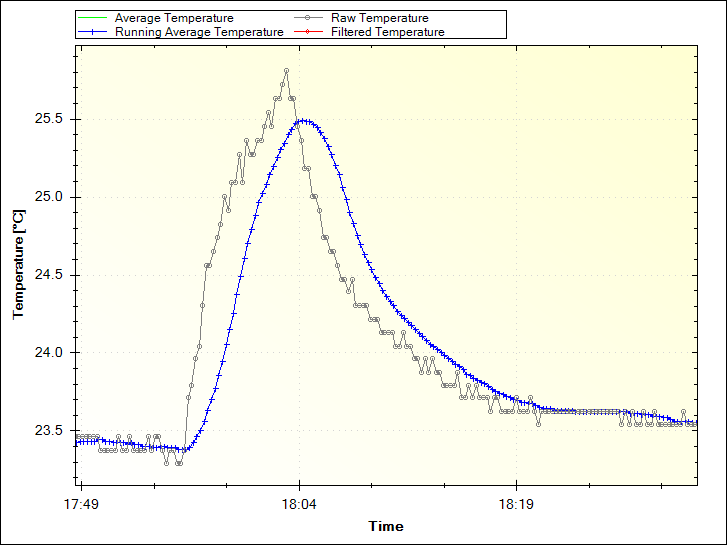A 16 point running average from a temperature sensor is plotted (blue). The raw temperature is shown in grey.

If the delay at the end of the loop was reduced from 100 ms to 1 ms, the response of the running average would be the same as the simple average. However, the longer delay between measurements is time when the Arduino could be asleep, saving battery power. Or busy doing something else.

The running average seems like a good alternative to a simple average to give a smoother output and let the Arduino work on other things. But it has one big down side: memory use.

Because you have to keep track of the history to calculate a running average, filtering many measurements quickly becomes impractical. The Arduino Uno only has 2k of RAM to store this history and you will quickly run out. If you needed to keep the history for some other reason, it could be a good choice. But I wouldn’t use a running average filter on an Arduino very often because of the amount of memory it uses.

### Exponential Filter

The last filter is a recursive filter. A recursive filter is just one that calculates a new, smoothed value (yn) by using the last smoothed value (yn – 1) and a new measurement (xn):

yn = w × xn + (1 – w) × yn – 1

The amount of smoothing is controlled by a weighting parameter (w). The weight is a value between 0% and 100%. When the weight is high (say 90%), the filter doesn’t smooth the measurements very much but responds quickly to changes. If the weight is low (say 10%), the filter smooths the measurements a lot but doesn’t respond very quickly to changes.

This is my favorite filter because:

• it doesn’t need much memory (just enough to store the last measurement)
• you can control how much filtering is applied with a single parameter (the weight)
• it works well in battery powered applications because you don’t need to make many measurements at once

Its so useful, we added the exponential filter to our Arduino library. And that makes it easy to use as well! Here’s what you need:

With a weight of 20, we get a fairly strong filter but it is still more responsive than the running average: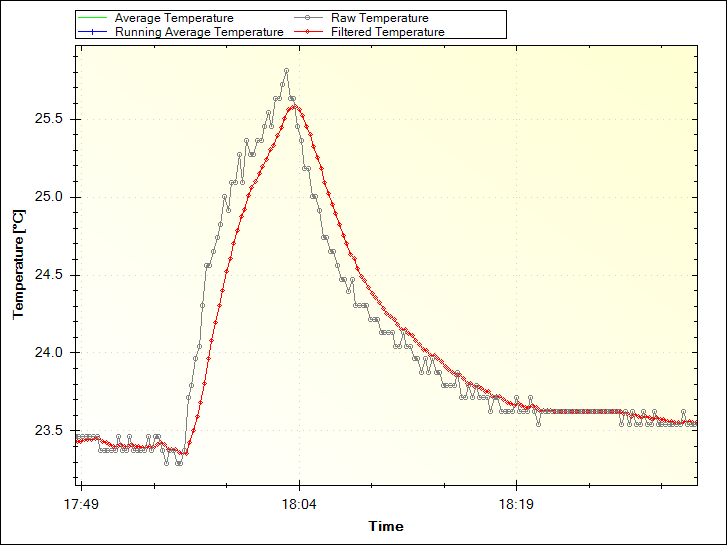Raw temperature measurements from a thermistor (grey) and a temperature measurement smoothed by an exponential filter (red) are shown.

## Conclusion

So there we have it. 3 methods to filter noisy measurements you make with an Arduino:

• Averaging: easy to implement and understand.
• Running average: can use a lot of memory; usually not a good choice for an Arduino sketch.
• Exponential filter: easy to change the amount of filtering using a weight; doesn’t need much memory; easy to implement with our Arduino filter library.

To test these filters, plot your raw data and the filtered measurements with MegunoLink to see how the filter responds when the data changes. Pick the one that gives the smoothest result without distorting the original data. Which one worked best for you? Post a comment below and let us know how you get on.

Check out our documentation for help getting started with MegunoLink plotting.

Recent Posts
• CodeStranger

HI Paul,

First, great article and an introduction to analog data filtering.

What might be a better method for doing the running average, and needing far less storage, would be Welford’s method: https://en.wikipedia.org/wiki/Algorithms_for_calculating_variance#Online_algorithm

In pseudo-code:

running_avg = (running_avg * (n-1))/n + rawTemp/n

Some care needs to be taken with wrapping n appropriately before it overflows. However, in this instance, you need to only store the running average (1 float), the count of measurements seen so far (1 int), and the rawTemp (1 float), or 10 bytes (assuming 4-byte floats and 2-byte ints).

• Paul Martinsen

Thanks. That’s a nice idea! That one would be an average of all the measurements seen so far, right?

Eventually, as n gets big, the contribution of rawTemp/n is going to be very small compared to running_avg*(n-1). Then changes in the temperature aren’t going to affect the average any more and we get a very accurate measure of the average signal.

• Alexander Ermoshenko

I use this methods for analog readings. May be they not best but I found it useful.

//////////////////////////////////////

/*
Expand range of reading to 15 bit. Values range [0..32736]
*/
{
int countSamples = 512;
long value = 0;
for(int i =0; i != countSamples; ++i)
{
}
return (int)(value / 16);
}
//////////////////////////////////////
// Using median if needed exact value of reading

void isort(int *a, int n)
{
for (int i = 1; i = 0) && (j > a[k]); k–)
{
a[k + 1] = a[k];
}
a[k + 1] = j;
}
}

{
for(int i =0; i != READ_MEDIAN_COUNT; ++i)
{
}
return values[READ_MEDIAN_COUNT / 2 + 1];
}

• Paul Martinsen

Thanks for your comment Alexander. I particularly like your median filter. That one is good for dealing with measurements that have a lot of spiky noise on them. In average or exponential filters, large spikes can really throw off the average.

• Alexander Ermoshenko

Just tried filter library. But after all I stop using it. Big disadvantage of of that library is lots of dependencies that increase binary size. And initialization of start value thru constructor without ability to reinitialize during work. But its not suited for me, because I need preheat sensors first. And need reinitialize filter after preheating is completed. I decided to write down my own class, and its very very simple.

// Exponential smoothing filter
// init with coeff, for me 0.05 is normal
// set initial value via setValue(some reading from sensor)
// in update loop call updateValue and folowing getValue for filtered

class Filter
{
private:
float _value;
const float _coeff;
public:
// coeff [0..1] if near to zero filter more inert
Filter(float coeff):
_coeff(coeff)
{
}

// set initial value
void setValue(float value)
{
_value = value;
}

//get filtered value
float getValue() const
{
return _value;
}
// update inernal value of filter
float updateValue(float value)
{
_value = value * _coeff + _value * (1 – _coeff);
return _value;
}
};

• Philip Rowe

Hi Alexander, nice work coming up with a solution that works for you. We’re a bit confused as the linker should strip out any of the unused parts reducing memory consumption. How did you measure the reduction in resources?
Cheers
Phil

• Kyle

Hello Paul,
Great intro to data smoothing, thank you.
I’m working on a project for a class of environmental science students that will require them to smooth raw data (they’ll actually be capturing raw data, and smoothing it after the fact). However, since the A/D converters on our Arduinos are only 10 bit, little to no noise is recorded from the sensors I’ve tried.
Can you tell me what model thermistor you used? Or if you know of any other sensors that are particularly noisy, that would also be of great help (I’ve probably just never bought a cheap enough one).

Thanks again!

• Philip Rowe

Hi Kyle, I chatted to Paul but we don’t have the details on the thermistor anymore. He suggested you get one with a large resistance which should be noiser. Not sure it would have the same effect (teaching tool wise) but you could also introduce random noise programmatically.

Cheers Phil

• dawoud

Hello :
Sorry; Do not you want to answer my question?
The measurement changes steadily and is not accurate.
Some friends told me that using the averaging method, the problem would be solved.
Is this true?
I would appreciate it if you guide me

• Philip Rowe

Hi, generally load cells typically drift over long periods. That’s why you need to zero food scales or bathroom scales. Filtering likely won’t help remove this slow drift.

• dawoud

Thank you:
What is your suggestion to solve the problem?

• gianmarco

i am experimenting with the exponential filter and the library provided

i have noticed a weird quirk, not sure it is a feature or a bug
if i initialize a filter using a low weight, say 10, and a low starting value, for instance 0, and do a number of readings of a x value, say 20, the filtered value never reach 20,

in this example the ADCFilter0.Current stabilizes on 11, while i would expect it to converge towards 20

#include

void setup() {
// put your setup code here, to run once:
Serial.begin(115200);
}

void loop() {
// put your main code here, to run repeatedly:

for (int i=0; i <= 1000; i++){

delay(10000);

}

if i set the initial value to an arbitrarily large number, say 1000, the ADCFilter0.Current() will slowly (and i assume correctly) converge to 20

• Philip Rowe

Good catch. Will look into a fix for this.
Phil

• gianmarco

thanks a lot, much appreciated.

• Galih Setyawan

Can i translate your article to me language and then i reference to your web?
.

• Philip Rowe

Yeah go for it. Let us know when its done and maybe we can link to it too.

Cheers
Phil

• Zaki

Halo philip and paul… i have some problems with my coding…i make a project using LM35 temperature sensor…this is my program:

int val;
int tempPin = 1;

void setup()
{
Serial.begin(9600);
}
void loop()
{
float mv = ( val/1024.0)*5000;
float cel = mv/10;

Serial.print(“TEMPRATURE = “);
Serial.print(cel);
Serial.print(“*C”);
Serial.println();
delay(1000);
—————————
that program will read sensor value every 1 second…but i want to get average of sensor value every 10 seconds (AVERAGE=(T1+T2+…T10)/10)….how i write that in the program paul and philip??i dont understand…